## 2.1.1 Problem Formulation

The discrete feasible planning model will be defined using state-space models, which will appear repeatedly throughout this book. Most of these will be natural extensions of the model presented in this section. The basic idea is that each distinct situation for the world is called a state, denoted by, and the set of all possible states is called a state space,. For discrete planning, it will be important that this set is countable; in most cases it will be finite. In a given application, the state space should be defined carefully so that irrelevant information is not encoded into a state (e.g., a planning problem that involves moving a robot in France should not encode information about whether certain light bulbs are on in China). The inclusion of irrelevant information can easily convert a problem that is amenable to efficient algorithmic solutions into one that is intractable. On the other hand, it is important thatis large enough to include all information that is relevant to solve the task.

The world may be transformed through the application of actions that are chosen by the planner. Each action,, when applied from the current state,, produces a new state,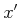, as specified by a state transition function,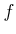. It is convenient to useto express a state transition equation,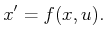(2.1)

Let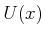denote the action space for each state, which represents the set of all actions that could be applied from. For distinct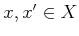,and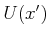are not necessarily disjoint; the same action may be applicable in multiple states. Therefore, it is convenient to define the set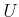of all possible actions over all states: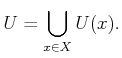(2.2)

As part of the planning problem, a set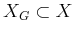of goal states is defined. The task of a planning algorithm is to find a finite sequence of actions that when applied, transforms the initial state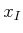to some state in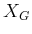. The model is summarized as:

Formulation 2..1 (Discrete Feasible Planning)
1. A nonempty state space, which is a finite or countably infinite set of states.
2. For each state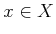, a finite action space.
3. A state transition functionthat produces a state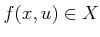for everyand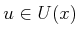. The state transition equation is derived fromas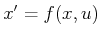.
4. An initial state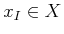.
5. A goal set.

It is often convenient to express Formulation 2.1 as a directed state transition graph. The set of vertices is the state space. A directed edge fromto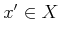exists in the graph if and only if there exists an actionsuch that. The initial state and goal set are designated as special vertices in the graph, which completes the representation of Formulation 2.1 in graph form.

Steven M LaValle 2020-08-14